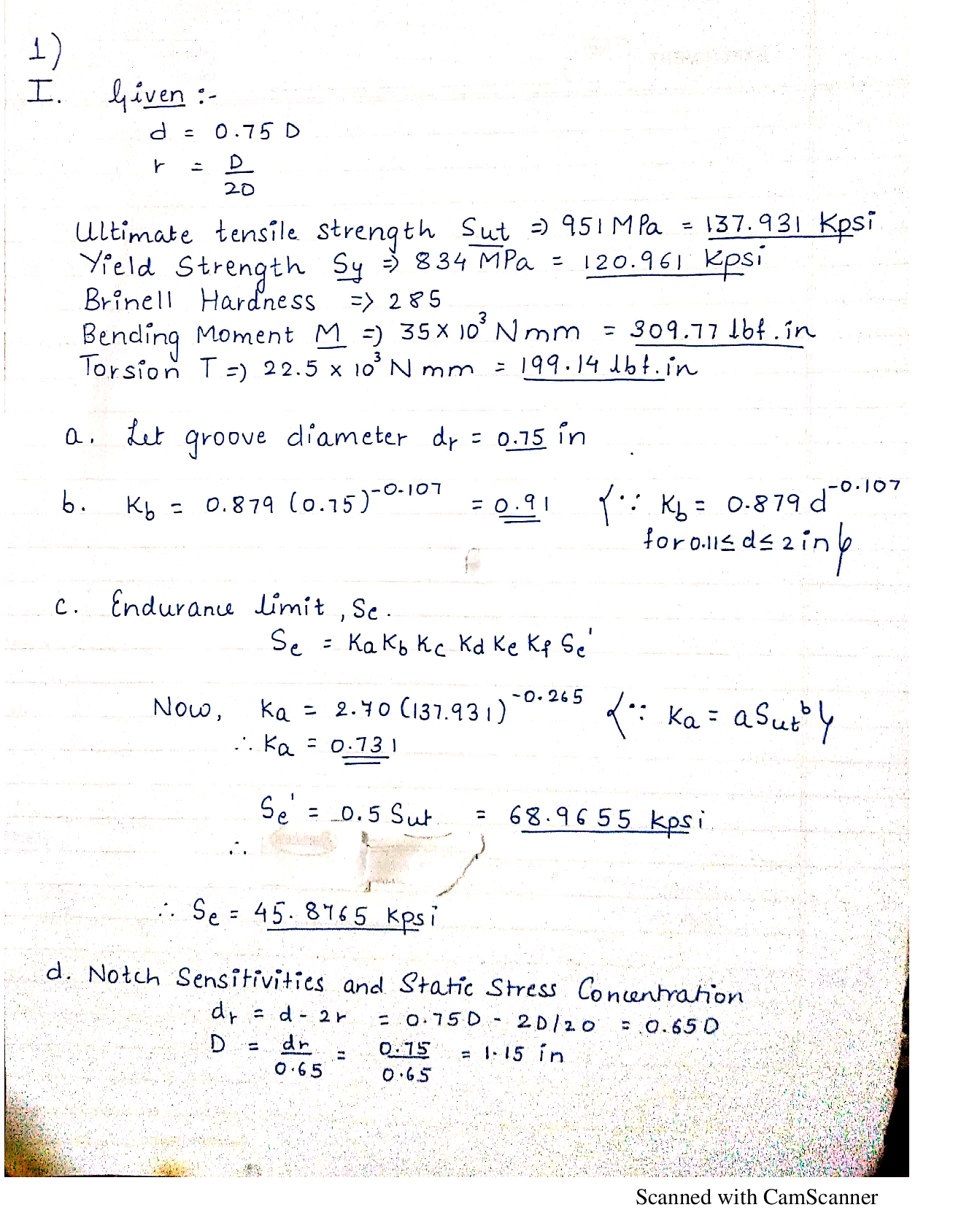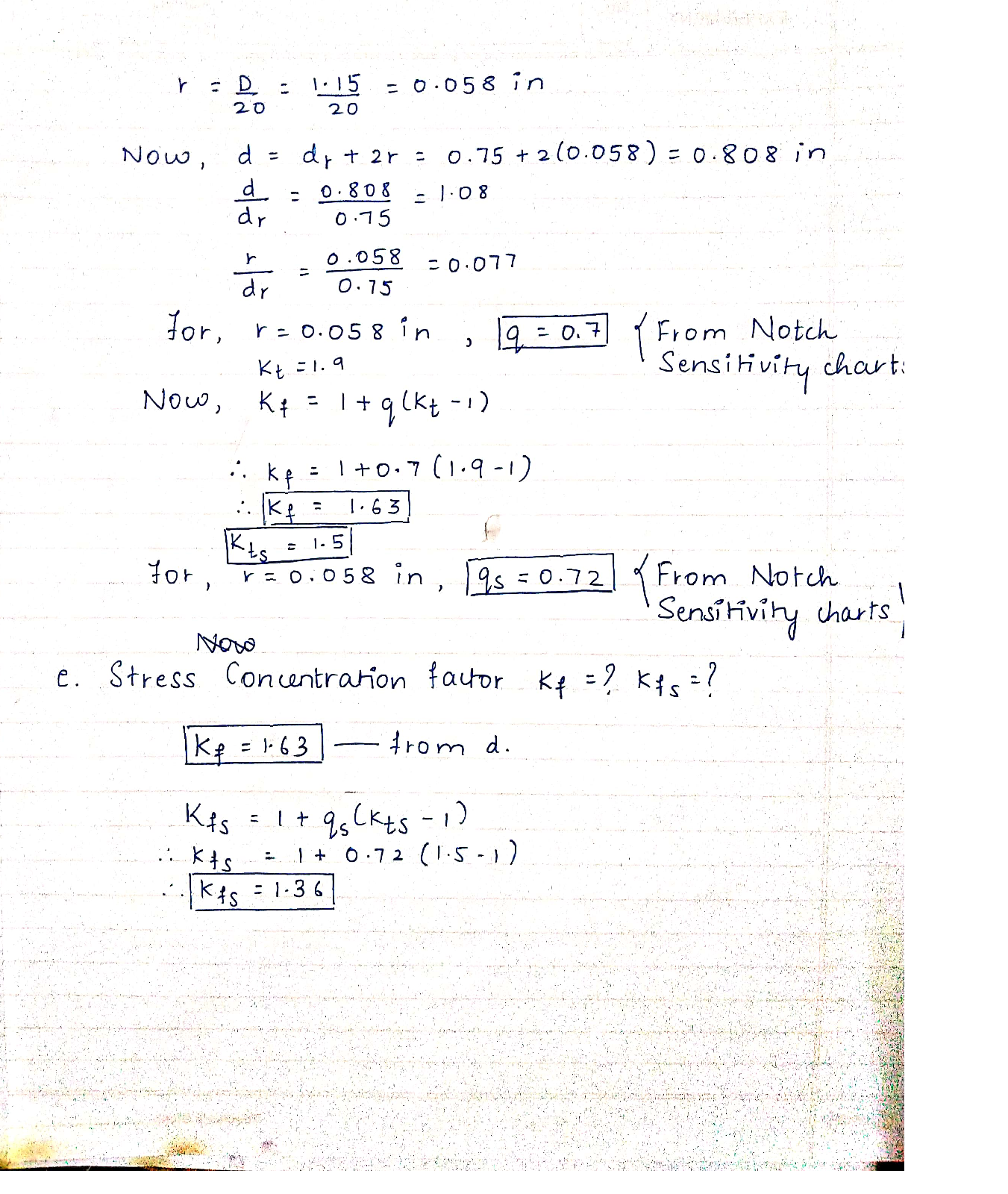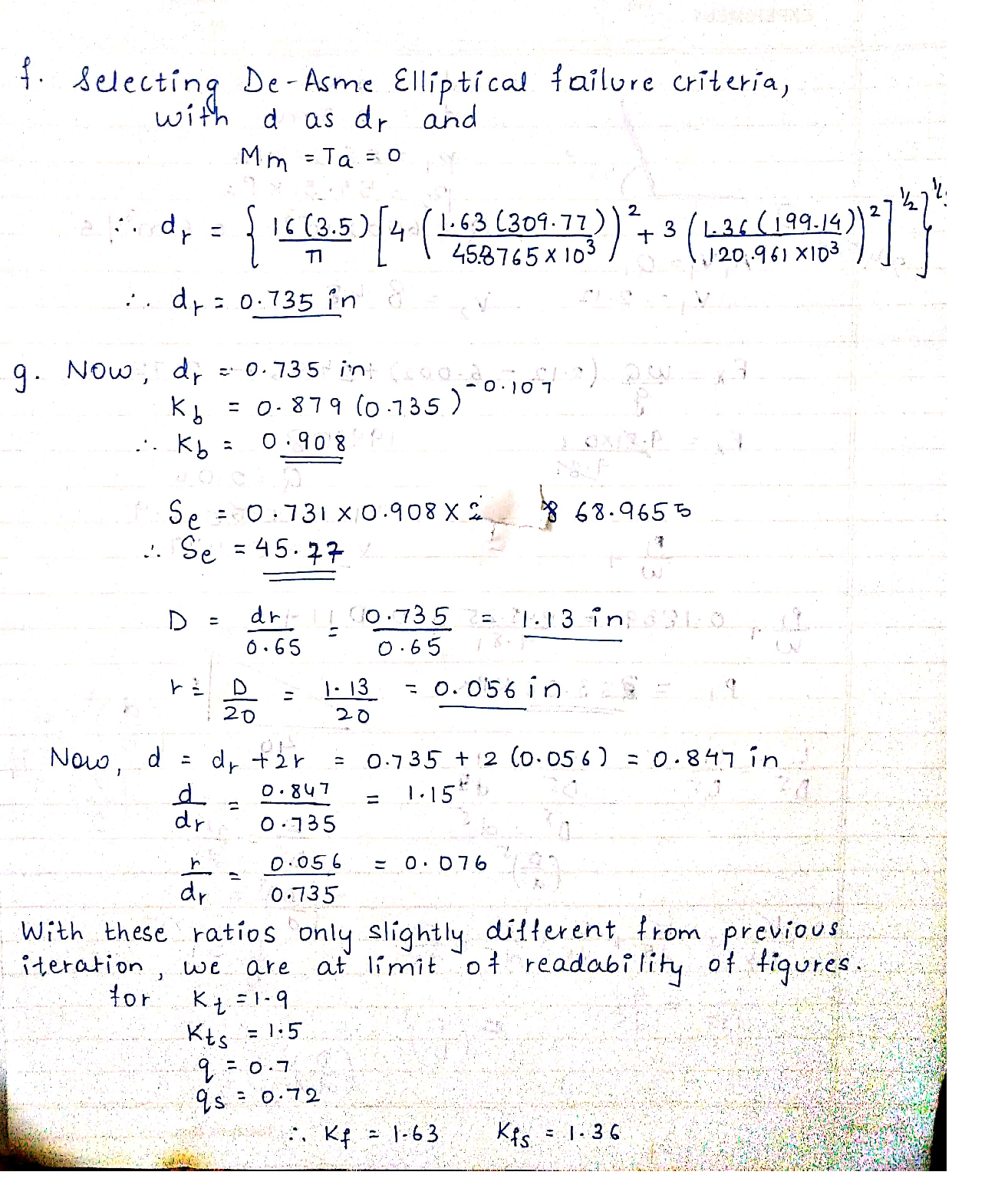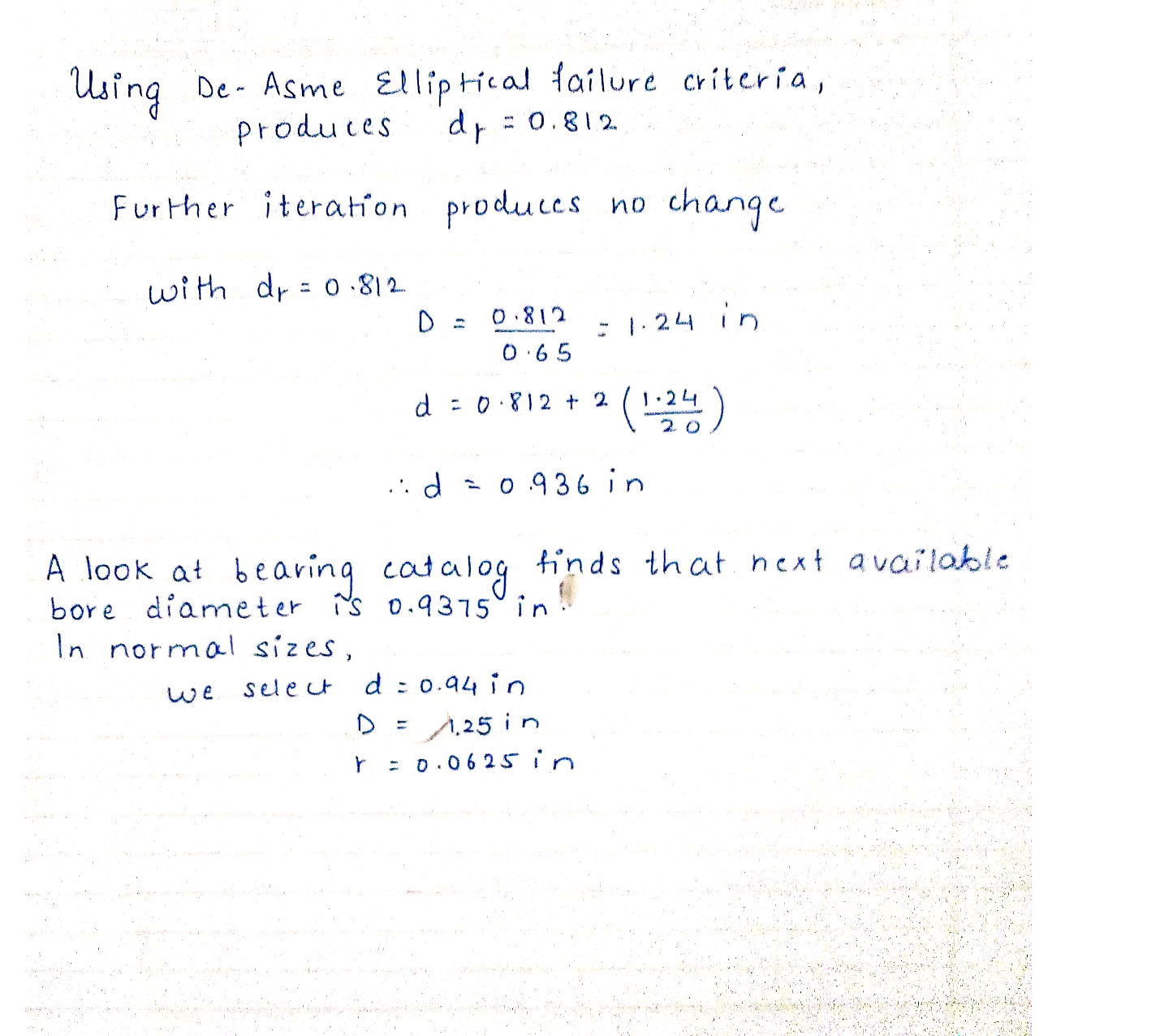Question

Fluid Mechanics

1) The section of shaft shown in the figure is to be designed to approximate relative sizes of d = 0.75D and r = D/20 with diameter d conforming to that of standard metric rolling-bearing bore sizes. The shaft is to be made of AISI 4140 steel, heat-treated to obtain minimum strengths in the shoulder area of 951 MPa ultimate tensile strength and 834 MPa yield strength with a Brinell hardness not less than285. At the shoulder the shaft is subjected to a completely reversed bending moment of 35 x 10 N.mm, accompanied by steady torsion of 22.5 x 103 N.mm.Surfaces are machined.

1. Choose a diameter at the root groove d, =?

b. Find the size factor, k, = ?

c. Find the Endurance limit S. = ?

d. Find the notch sensitivities and static stress concentration factors

q=?, q_{s}=?, K_{t}=?, K_{t s}=?

Find the fatigue stress concentration factors Kf = ?,Kfs = ?

Using a fatigue factor of safety according ASME-EIliptic, n =nASME = 3.5, estimate the diameter at the root groove, d,

d=\left\{\frac{16 n}{\pi}\left[4\left(\frac{K_{f} M_{a}}{S_{e}}\right)^{2}+3\left(\frac{K_{f s} T_{a}}{S_{e}}\right)^{2}+4\left(\frac{K_{f} M_{m}}{S_{y}}\right)^{2}+3\left(\frac{K_{f s} T_{m}}{S_{y}}\right)^{2}\right]^{1 / 2}\right\}^{1 / 3}

g. With this new calculated, d,, Loop through part b to part f, until you have a converged solution for the diameter at the root groove.Verified### Question 45484Fluid Mechanics

Water in the Belle Fourche Reservoir in Western South Dakotais transported to the water storage reservoir for the town of-Buffalo through a series of three pipes, all with friction factors of 0.02. The first pipe is 500 meters long with a diameter of 0.4meters; the second pipe is 600 meters long with a diameter of0.3 meters; and the third pipe is 500 meters long with a diameter of 0.2 meters. The water surface elevation of the Belle FourcheReservoir is 30 meters higher than the town's storage reservoir.Since the current piping is almost 60 years old, the town of Buffalo has decided to replace the piping system with a single pipe of constant diameter. You have been chosen to calculate the equivalent diameter for the single pipe design and determine the flow rate that will occur. Go to work.

### Question 44899Fluid Mechanics

Transmission lines

### Question 44896Fluid Mechanics

A boat has a volume of 40.0 m³ and a mass of 2.00 tons. What load will push 75.0% of its volume into water? Each Metric ton is 1000 kg.

### Question 44895Fluid Mechanics

A tank has a height of 800 mm and a length of 500 mm. The tank contains a liquid of RD 0.9.Determine:
a. The magnitude of the force on the side of the tank per meter width and its position relative to the base of the tank
b. The force on the base per meter width of tank.

### Question 44894Fluid Mechanics

A closed tank contains three different fluids with a vapor space above the oil, as shown in the figure below. Pressure gauge 1 reads the gauge pressure of the vapor space (P1) and pressure gauge 2 reads the pressure at the bottom of the tank (P2). When P1 = 25 kPa, determine:
a. Height of glycerine (h) in the tank if P2 = 95.66 kPa
. P2 reading if glycerine is replaced with water

### Question 44805Fluid Mechanics

Water flows into cylindrical tank through inlet A and D at rate of 20 ft/s and 5 ft/s respectively. If waterleaves through outlet B and C at rate of 3 ft/s and 10 ft/s respectively.
(A) Derive the continuity equation
\text { Know that } D_{A}=2.5 \text { in, } D_{D}=2 \text { in } D_{B}=3 \text { in and } D_{C}=3.5 \text { in. }
(B) Calculate the change of high h?

### Question 44804Fluid Mechanics

An object which has cubic shape is been floating on the water surface see the diagram below. If the object density is less than water, density find the ratio X/L?

### Question 44803Fluid Mechanics

A force of 15.83 kN is exerted on a piston B (diameter = 250 mm) which fits into a cylinder see the diagram below. How much force is need on piston C to start displacing piston B and Mercury on piston B?

### Question 44802Fluid Mechanics

Given the diagram below if the pressure gauge reads 6.1 psig find Y and X in ft. Given that
\mathrm{SG}_{\mathrm{Aloohal}}=0.9, \mathrm{SG}_{\mathrm{Hg}}=13.6, \text { Alcohol vapor } P=1.7 \mathrm{psig}, Z=4 \mathrm{ft} \text { and } \mathrm{Y}_{\mathrm{H} 2 \mathrm{o}}=62.4 \mathrm{\textrm {lb }} / \mathrm{ft}^{3}

### Question 44801Fluid Mechanics

Given the diagram below, calculate the pressure at A, B, C.
\text { Given that } \mathrm{Y} \text { н2о }=9.79 \mathrm{kN} / \mathrm{m}

### Submit query